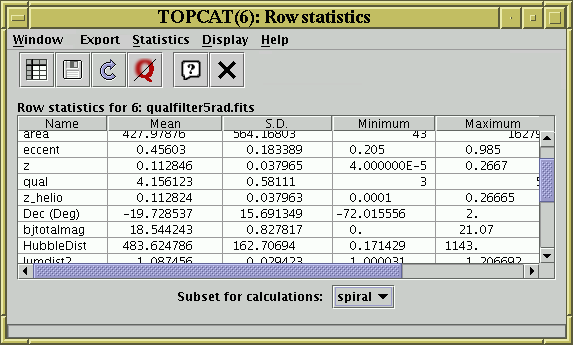Next Previous Up Contents
Up: Table View Windows
Previous: Subsets Window

### A.3.5 Statistics WindowStatistics Window

The Statistics Window shows statistics for the values in each of the table's columns. You can display it using the Column Statistics () button when the chosen table is selected in the Control Window's Table List.

The calculated values are displayed in a JTable widget with a row for each column in the main table, and a column for each of a number of statistical quantities calculated on some or all of the values in the data table column corresponding to that grid row.

You can interact with this JTable in the usual ways, for instance dragging columns sideways, changing their widths, and sorting the entries by clicking on the headings.

The following columns are shown by default:

Name
The name of the column in the main table represented by this grid row.
Mean
The mean value of the good cells. For boolean columns, this is the proportion of good cells which are True.
SD
The population standard deviation of the good cells.
Minimum
The minimum value. For numeric columns the meaning of this is quite obvious. For other columns, if an ordering can be reasonably defined on them, the 'smallest' value may be shown. For instance string values will show the entry which would be first alphabetically.
Maximum
As minimum, but shows the largest values.
nGood
The number of non-blank cells.
Several additional items of statistical information are also calculated, but the columns displaying these are hidden by default to avoid clutter. You can reveal these by using the Display menu:
Index
The index of the column in the table, i.e. the order in which it is displayed.
\$ID
The unique identifier label for the column in the main table.
Sum
The sum of all the values in the column. For boolean columns this is a count of the number of True values in the column.
Variance
The population variance of the good cells.
Sample SD
The sample standard deviation of the good cells.
Sample Variance
The sample variance of the good cells.
Median Absolute Deviation
The median of absolute deviations from the median: `median(abs(x-median(x))`. This is a robust measure of statistical dispersion.
Scaled Median Absolute Deviation
The Median Absolute Deviation (see above) multiplied by 1.4826. This is supposed to be a consistent estimator for the standard deviation, on the assumption of a normal distribution.
Skew
Gamma 1 measure of skewness of the value distribution.
Kurtosis
Gamma 2 measure of peakedness of the value distribution.
Row of min
The index of the row in the main table at which the minimum value occurred.
Row of max
The index of the row in the main table at which the maximum value occurred.
The number of blank cells; the sum of this value and the Good cells value will be the same for each column.
Cardinality
If the column contains a small number of distinct values then that number, the column's cardinality will be shown here. Cardinality is the number of distinct values which appear in that column. If the number of values represented is large (currently >50) or a large proportion of the non-bad values (currently >75%) then no value is shown.

In addition, some quantile values can calculated on demand (by selecting their values in the Display menu, as for the previous list). The available values are:

Q001:
value below which 0.1% of rows fall
Q01:
value below which 1% of rows fall (1st percentile)
Quartile1:
value below which 25% of rows fall (first quartile)
Median:
value below which 50% of rows fall (median)
Quartile3:
value below which 75% of rows fall (third quartile)
Q99:
value below which 99% of rows fall (99th percentile)
Q999:
value below which 99.9% of rows fall
These are considerably more expensive to calculate than the other statistical quantities, and so they are not provided by default. If you attempt to calculate them for large tables, you may get a message saying that there is insufficient memory.

The quantities displayed in this window are not necessarily those for the entire table; they are those for a particular Row Subset. At the bottom of the window is the Subset For Calculations selector, which allows you to choose which subset you want the calculations to be done for. By clicking on this you can calculate the statistics for different subsets. When the window is first opened, or when it is invoked from a menu or the toolbar in the Control Window, the subset will correspond to the current row subset.

The toolbar contains the following extra buttons:Save as Table
Clicking this button will save the quantities displayed in this window to a table on disk. It can be saved in any of the tabular formats which TOPCAT understands.Import as Table
The table of statistical quantities displayed by this window (rows corresponding to input table columns and columns corresponding to statistical quantities) is itself a table. By clicking this button it can be loaded into TOPCAT as a new table and manipulated in all the usual ways. This has the same effect as saving the statistics to file (see previous button) and then reloading that file.Recalculate
Once statistics have been calculated for a given subset they are cached and not normally recalculated again. Use this button if you want to force a recalculation because the data may have changed.

For a large table the calculations may take a little while. While they are being performed you can interact with the window as normal, but a progress bar is shown at the bottom of the window. If you initiate a new calculation (by pushing the Recalculate button or selecting a new subset) or close the window during a calculation, the superceded calculation will be stopped.

Next Previous Up Contents